## Example Questions

### Example Question #1 : Percentage

What percentage of a solution is blood if it contains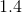ml blood and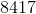ml water?  Round to the nearest thousandth?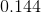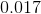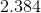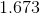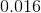Explanation:

First, you must find the total amount of solution.  This is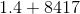, or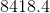.

Now, the percentage of the solution that is blood can be represented: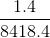, or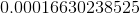This is the same as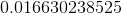%  Rounded, it is%

### Example Question #2 : Percentage

What is the percentage increase of an account that began with a balance of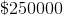and increased to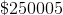?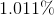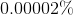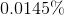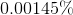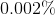Explanation:

Recall that the formula for percentage increase is: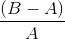, whereis your ending value andis your starting value.

For our numbers, this would be: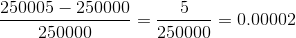Recall that to find a percentage from a decimal, you multiply by: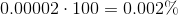Tired of practice problems?

Try live online GRE prep today.